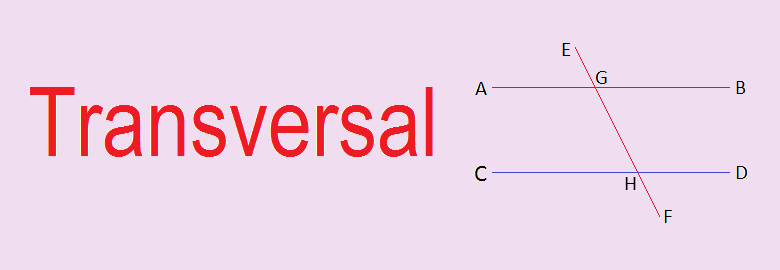# Transversal## Transversal

In geometry, a transversal is a line which cuts two or more lines at distinct points. In the figure given below, lines AB and CD are cut by the line EF at the points G and H respectively. So, EF is a transversal.

### Types of angles formed by a transversal

When two line segments are cut by a transversal then there will form 8 angles. In the figure given above, transversal EF has cut the line segments AB and CD at points G and H respectively. The angles formed there are AGE, BGE, AGH, BGH, GHC, GHD, CHF and DHF.
Interior angles: The angles formed inside the two line segments are called interior angles. In the given figure, angles AGH, BGH, GHC and GHD are interior angles.

Exterior angles: The angles formed outside the two line segments are called exterior angles. In the given figure, angles AGE, BGE, CHF and DHF are exterior angles.

#### Alternate angles

A pair of non adjacent interior angles on the opposite sides of a transversal is called alternate angles
In the figure the alternate angles are:
i.      AGH and GHD
ii.    BGH and GHC
Note: When the two lines cut by transversal are parallel, then alternate angles are equal.

#### Corresponding angles

In a pair of non-adjacent angles on the same side of a transversal, one is external and other internal are called corresponding angles.
In the figure the corresponding angles are:
i.      AGE and GHC
ii.    AGH and CHF
iii.   BGE and GHD
iv.   BGH and DHF
Note: When the two lines cut by transversal are parallel, then corresponding angles are equal.

#### Co-interior angles

A pair of two interior angles on the same side of a transversal are called co-interior angles.
In the figure the co-interior angles are:
i.      AGH and GHC
ii.     BGH and GHD
Note: When the two lines cut by transversal are parallel, then co-interior angles are supplementary i.e. their sum is 180°.

### Workout Examples

Example 1: Find the values of a, b, c and d from the given figure.
Solution: Here,
5a + 30° + 3a – 10° = 180° ----------------> sum of co-interior angles
or,     8a + 20° = 180°
or,     8a = 180° - 20°
or,     a = 160°/8
or,     a = 20°

b = 5a + 30° --------------------> Corresponding angles
= 5×20° + 30°
= 100° + 30°
= 130°

c = 3a – 10° --------------------> Alternate angles
= 3×20° – 10°
= 60° – 10°
= 50°

d = c ----------------------> Vertically opposite angles
= 50°

a = 20°, b = 130°, c = 50° and d = 50°

You can comment your questions or problems regarding parallel lines and transversal here.